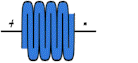# The Electrostatic Muscle

.

## The Basic Idea

Next to the fields holding atomic nucleus together, the electrostatic field is the strongest in nature.   The electrostatic muscle uses this force to power the contraction of an accordion-like structure:

The blue filling is an elastic dielectric.  The black is aluminum foil.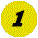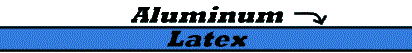Start with a layer of latex sandwiched between two layers of aluminum foil.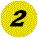Z-fold the sandwich and...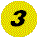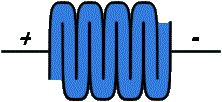Apply a power supply to your device and you have a synthetic muscle.

If you have a single flat capacitor-like structure (with an elastic dielectric) that contracts when a voltage is applied you will get some small displacement.   If for example, you have a separation of .001 M that will contract by 50% when some voltage is applied.  The contraction will yield a new thickness of .0005 M.   When this contraction is multiplied by some number of concatenated layers you get a contracted thickness of 50% of the uncontracted length multiplied by the number of layers.   So, if you have 1,000 layers this gives your folded thickness a length of .001M*1,000 or 1M.  The contracted length then is .5M.

## The Force

If the force between two layers is, for example, .001 Newtons, then, for 1000 layers you get a force of 1 Newton.  You can increase the force by either increasing the number of layers, increasing the plate charge or by reducing the thickness of the layers.

Copyright 1998, by Jack Martinelli